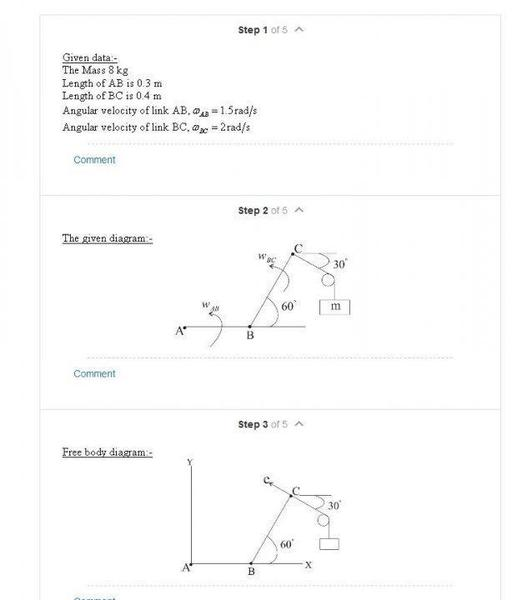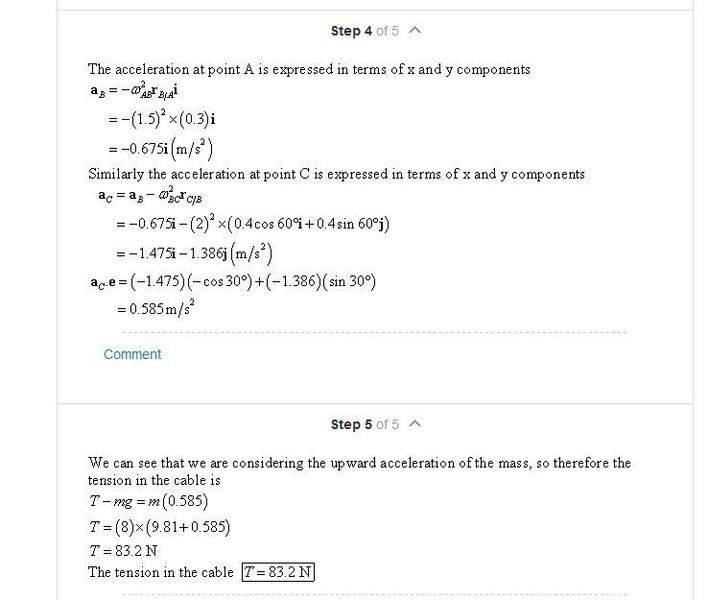# Understanding solution method for finding accelerations in a mechanical linkage

• whitejac

## Homework Statement

I was checking my work and Chegg uses the equations differently. Can somebody tell me why? Maybe I'm misunderstanding how/why to use the equation I chose.## Homework Equations

They say aB = -ω2ABRB/Ai
I used aB = aA + αk x r - ω2rB/A

## The Attempt at a Solution

So obviously mine will be a different answer, I only found aB but it gave me an i and j component because of that cross product. Which one is correct and why? Why can they neglect the cross product?

The cross product term involves angular acceleration of the rod connecting A and B. In the Chegg problem, the angular acceleration is apparently zero.

How do they justify there being no angular acceleration? It doesn't say this is a static situation, so these bars should be rotating towards one another.

How do they justify there being no angular acceleration? It doesn't say this is a static situation, so these bars should be rotating towards one another.

I figured it out. The problem states that the velocities are constant and therefore the angular accelerations are zero.
Man, reading is important. Thank you for explaining this to me!